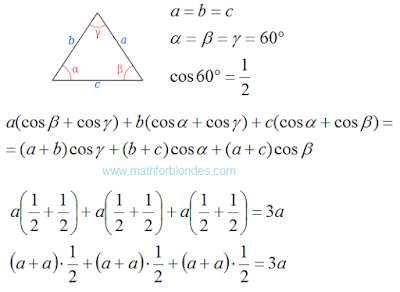## 3.15.2016

### Check the law of cosines

Check the law of cosines for the perimeter, we start with an equilateral triangle. All sides of an equilateral triangle are equal. All angles are equal to 60 degrees. The perimeter of the triangle is equal to three times the length of the sides.Equilateral triangle

Equality is performed. Now we check the law of cosines in a right triangle.